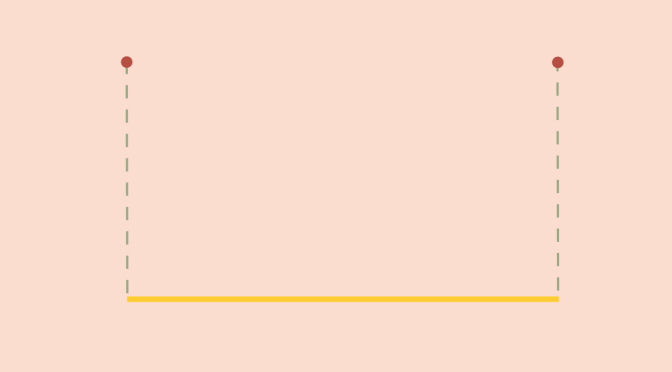A discontinuous real convex function

Consider a function $$f$$ defined on a real interval $$I \subset \mathbb R$$. $$f$$ is called convex if: $\forall x, y \in I \ \forall \lambda \in [0,1]: \ f((1-\lambda)x+\lambda y) \le (1-\lambda) f(x) + \lambda f(y)$

Suppose that $$I$$ is a closed interval: $$I=[a,b]$$ with $$a < b$$. For $$a < s < t < u < b$$ one can prove that: $\frac{f(t)-f(s)}{t-s}\le \frac{f(u)-f(s)}{u-s}\le\frac{f(u)-f(t)}{u-t}.$ It follows from those relations that $$f$$ has left-hand and right-hand derivatives at each point of the interior of $$I$$. And therefore that $$f$$ is continuous at each point of the interior of $$I$$.
Is a convex function defined on an interval $$I$$ continuous at all points of the interval? That might not be the case and a simple example is the function: $\begin{array}{l|rcl} f : & [0,1] & \longrightarrow & \mathbb R \\ & x & \longmapsto & 0 \text{ for } x \in (0,1) \\ & x & \longmapsto & 1 \text{ else}\end{array}$

It can be easily verified that $$f$$ is convex. However, $$f$$ is not continuous at $$0$$ and $$1$$.